## Nuclear Equations

### Learning Objectives

By the end of this module, you will be able to:

• Identify common particles and energies involved in nuclear reactions
• Write and balance nuclear equations

Changes of nuclei that result in changes in their atomic numbers, mass numbers, or energy states are nuclear reactions. To describe a nuclear reaction, we use an equation that identifies the nuclides involved in the reaction, their mass numbers and atomic numbers, and the other particles involved in the reaction.

## Types of Particles in Nuclear Reactions

Many entities can be involved in nuclear reactions. The most common are protons, neutrons, alpha particles, beta particles, positrons, and gamma rays, as shown in Table 1. Protons $\left({}_{1}^{1}\text{p}\text{, also represented by the symbol }{}_{1}^{1}\text{H}\right)$ and neutrons $\left({}_{0}^{1}\text{n}\right)$ are the constituents of atomic nuclei, and have been described previously. Alpha particles $\left({}_{2}^{4}\text{He}\text{, also represented by the symbol }_{2}^{4}\alpha\right)$ are high-energy helium nuclei. Beta particles $\left({}_{-1}^{0}\beta\text{, also represented by the symbol }_{-1}^{0}\text{e}\right)$ are high-energy electrons, and gamma rays are photons of very high-energy electromagnetic radiation. Positrons $\left({}_{+1}^{0}\beta\text{, also represented by the symbol }_{+1}^{0}\beta\right)$ are positively charged electrons (“anti-electrons”). The subscripts and superscripts are necessary for balancing nuclear equations, but are usually optional in other circumstances. For example, an alpha particle is a helium nucleus (He) with a charge of +2 and a mass number of 4, so it is symbolized ${}_{2}^{4}\text{He}$. This works because, in general, the ion charge is not important in the balancing of nuclear equations.

Although many species are encountered in nuclear reactions, Table 1 summarizes the names, symbols, representations, and descriptions of the most common of these.

Table 1. Nuclear Particles
Name Symbol(s) Representation Description
Alpha particle ${}_{2}^{4}\text{He}$  or  ${}_{2}^{4}\alpha$(High-energy) helium nuclei consisting of two protons and two neutrons
Beta particle ${}_{-1}^{0}\text{e}$  or  ${}_{-1}^{0}\beta$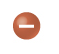(High-energy) electrons
Positron ${}_{+1}^{0}\text{e}$  or  ${}_{+1}^{0}\beta$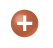Particles with the same mass as an electron but with 1 unit of positive charge
Proton  ${}_{1}^{1}\text{H}$  or  ${}_{1}^{1}\text{p}$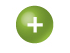Nuclei of hydrogen atoms
Neutron  ${}_{0}^{1}\text{n}$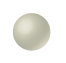Particles with a mass approximately equal to that of a proton but with no charge
Gamma ray  $\gamma$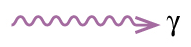Very high-energy electromagnetic radiation

Note that positrons are exactly like electrons, except they have the opposite charge. They are the most common example of antimatter, particles with the same mass but the opposite state of another property (for example, charge) than ordinary matter. When antimatter encounters ordinary matter, both are annihilated and their mass is converted into energy in the form of gamma rays (γ)—and other much smaller subnuclear particles, which are beyond the scope of this chapter—according to the mass-energy equivalence equation E = mc2, seen in the preceding section. For example, when a positron and an electron collide, both are annihilated and two gamma ray photons are created:

${}_{-1}^{0}\text{e}+{}_{+1}^{0}\text{e}\longrightarrow \gamma+\gamma$

As seen in the chapter discussing light and electromagnetic radiation, gamma rays compose short wavelength, high-energy electromagnetic radiation and are (much) more energetic than better-known X-rays that can behave as particles in the wave-particle duality sense. Gamma rays are produced when a nucleus undergoes a transition from a higher to a lower energy state, similar to how a photon is produced by an electronic transition from a higher to a lower energy level. Due to the much larger energy differences between nuclear energy shells, gamma rays emanating from a nucleus have energies that are typically millions of times larger than electromagnetic radiation emanating from electronic transitions.

## Balancing Nuclear Reactions

A balanced chemical reaction equation reflects the fact that during a chemical reaction, bonds break and form, and atoms are rearranged, but the total numbers of atoms of each element are conserved and do not change. A balanced nuclear reaction equation indicates that there is a rearrangement during a nuclear reaction, but of subatomic particles rather than atoms. Nuclear reactions also follow conservation laws, and they are balanced in two ways:

1. The sum of the mass numbers of the reactants equals the sum of the mass numbers of the products.
2. The sum of the charges of the reactants equals the sum of the charges of the products.

If the atomic number and the mass number of all but one of the particles in a nuclear reaction are known, we can identify the particle by balancing the reaction. For instance, we could determine that ${}_{8}^{17}\text{O}$ is a product of the nuclear reaction of ${}_{7}^{14}\text{N}$ and ${}_{2}^{4}\text{He}$ if we knew that a proton, ${}_{1}^{1}\text{H}$, was one of the two products. Example 1 shows how we can identify a nuclide by balancing the nuclear reaction.

### Example 1: Balancing Equations for Nuclear Reactions

The reaction of an α particle with magnesium-25 $\left({}_{12}^{25}\text{Mg}\right)$ produces a proton and a nuclide of another element. Identify the new nuclide produced.

The nuclide ${}_{53}^{125}\text{I}$ combines with an electron and produces a new nucleus and no other massive particles. What is the equation for this reaction?

Following are the equations of several nuclear reactions that have important roles in the history of nuclear chemistry:

• The first naturally occurring unstable element that was isolated, polonium, was discovered by the Polish scientist Marie Curie and her husband Pierre in 1898. It decays, emitting α particles:
• ${}_{84}^{212}\text{Po}\longrightarrow {}_{82}^{208}\text{Pb}+{}_{2}^{4}\text{He}$
• The first nuclide to be prepared by artificial means was an isotope of oxygen, 17O. It was made by Ernest Rutherford in 1919 by bombarding nitrogen atoms with α particles:
• ${}_{7}^{14}\text{N}+{}_{2}^{4}\alpha\longrightarrow {}_{8}^{17}\text{O}+{}_{1}^{1}\text{H}$
• James Chadwick discovered the neutron in 1932, as a previously unknown neutral particle produced along with 12C by the nuclear reaction between 9Be and 4He:
• ${}_{4}^{9}\text{Be}+{}_{2}^{4}\text{He}\longrightarrow {}_{6}^{12}\text{C}+{}_{0}^{1}\text{n}$
• The first element to be prepared that does not occur naturally on the earth, technetium, was created by bombardment of molybdenum by deuterons (heavy hydrogen, ${}_{1}^{2}\text{H}\)$ , by Emilio Segre and Carlo Perrier in 1937:
• ${}_{1}^{2}\text{H}+{}_{42}^{97}\text{Mo}\longrightarrow 2{}_{0}^{1}\text{n}+{}_{43}^{97}\text{Tc}$
• The first controlled nuclear chain reaction was carried out in a reactor at the University of Chicago in 1942. One of the many reactions involved was:
• ${}_{92}^{235}\text{U}+{}_{0}^{1}\text{n}\longrightarrow {}_{35}^{87}\text{Br}+{}_{57}^{146}\text{La}+3{}_{0}^{1}\text{n}$.

### Key Concepts and Summary

Nuclei can undergo reactions that change their number of protons, number of neutrons, or energy state. Many different particles can be involved in nuclear reactions. The most common are protons, neutrons, positrons (which are positively charged electrons), alpha (α) particles (which are high-energy helium nuclei), beta (β) particles (which are high-energy electrons), and gamma (γ) rays (which compose high-energy electromagnetic radiation). As with chemical reactions, nuclear reactions are always balanced. When a nuclear reaction occurs, the total mass (number) and the total charge remain unchanged.

### Exercises

Write a brief description or definition of each of the following:

1. nucleon
2. α particle
3. β particle
4. positron
5. γ ray
6. nuclide
7. mass number
8. atomic number

### Exercises

1. Which of the various particles (α particles, β particles, and so on) that may be produced in a nuclear reaction are actually nuclei.
2. Complete each of the following equations by adding the missing species:
1. ${}_{13}^{27}\text{Al}+{}_{2}^{4}\text{He}\longrightarrow ?+{}_{0}^{1}\text{n}$
2. ${}_{94}^{239}\text{Pu}+?\longrightarrow {}_{96}^{242}\text{Cm}+{}_{0}^{1}\text{n}$
3. ${}_{7}^{14}\text{N}+{}_{2}^{4}\text{He}\longrightarrow \text{?}+{}_{1}^{1}\text{H}$
4. ${}_{92}^{235}\text{U}\longrightarrow \text{?}+{}_{55}^{135}\text{Cs}+4{}_{0}^{1}\text{n}$
3. Complete each of the following equations:
1. ${}_{3}^{7}\text{Li}+\text{?}\longrightarrow 2{}_{2}^{4}\text{He}$
2. ${}_{6}^{14}\text{C}\longrightarrow {}_{7}^{14}\text{N}+\text{?}$
3. ${}_{13}^{27}\text{Al}+{}_{2}^{4}\text{He}\longrightarrow \text{?}+{}_{0}^{1}\text{n}$
4. ${}_{96}^{250}\text{Cm}\longrightarrow \text{?}+{}_{38}^{98}\text{Sr}+4{}_{0}^{1}\text{n}$
4. Write a balanced equation for each of the following nuclear reactions:
1. the production of 17O from 14N by α particle bombardment
2. the production of 14C from 14N by neutron bombardment
3. the production of 233Th from 232Th by neutron bombardment
4. the production of 239U from 238U by ${}_{1}^{2}\text{H}$ bombardment
5. Technetium-99 is prepared from 98Mo. Molybdenum-98 combines with a neutron to give molybdenum-99, an unstable isotope that emits a β particle to yield an excited form of technetium-99, represented as 99Tc*. This excited nucleus relaxes to the ground state, represented as 99Tc, by emitting a γ ray. The ground state of 99Tc then emits a β particle. Write the equations for each of these nuclear reactions.
6. The mass of the atom ${}_{9}^{19}\text{F}$ is 18.99840 amu.
1. Calculate its binding energy per atom in millions of electron volts.
2. Calculate its binding energy per nucleon.
7. For the reaction ${}_{6}^{14}\text{C}\longrightarrow {}_{7}^{14}\text{N}+\text{?}$, if 100.0 g of carbon reacts, what volume of nitrogen gas (N2) is produced at 273K and 1 atm?

## Glossary

alpha particle: (α or ${}_{\mathbf{2}}^{\mathbf{4}}\mathbf{\text{He}}$ or ${}_{\mathbf{2}}^{\mathbf{4}}\mathbf{\alpha})$ high-energy helium nucleus; a helium atom that has lost two electrons and contains two protons and two neutrons

antimatter: particles with the same mass but opposite properties (such as charge) of ordinary particles

beta particle: $\mathbf\beta$ or ${}_{\mathbf{-1}}^{\mathbf{0}}\mathbf{\text{e}}$ or ${}_{\mathbf{-1}}^{\mathbf{0}}\mathbf{\beta}\mathbf{\text{)}}$ high-energy electron

gamma ray: (γ or ${}_{\mathbf{0}}^{\mathbf{0}}\mathbf{\gamma}\mathbf{\text{)}}$ short wavelength, high-energy electromagnetic radiation that exhibits wave-particle duality

nuclear reaction: change to a nucleus resulting in changes in the atomic number, mass number, or energy state

positron $\text{(}{}_{+1}^{0}\beta_{\phantom{}}^{\phantom{}}$ or ${}_{+1}^{0}\text{e}\text{)}$ : antiparticle to the electron; it has identical properties to an electron, except for having the opposite (positive) charge